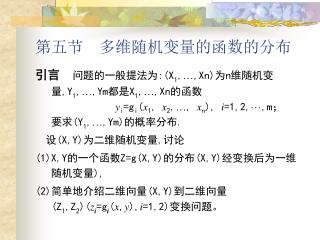DownloadDownload Presentation# 第五节 多维随机变量的函数的分布

Download Presentation## 第五节 多维随机变量的函数的分布

- - - - - - - - - - - - - - - - - - - - - - - - - - - E N D - - - - - - - - - - - - - - - - - - - - - - - - - - -
##### Presentation Transcript

1. 第五节 多维随机变量的函数的分布 引言 问题的一般提法为:(X1,…,Xn)为n维随机变量,Y1,…,Ym都是X1,…,Xn的函数yi=gi(x1, x2,…, xn), i=1,2,···,m；要求(Y1,…,Ym)的概率分布. 设(X,Y)为二维随机变量,讨论 (1)X,Y的一个函数Z=g(X,Y)的分布(X,Y)经变换后为一维随机变量), (2)简单地介绍二维向量(X,Y)到二维向量(Z1,Z2)(zi=gi(x,y),i=1,2)变换问题。

2. 0 1 2 3 4 5 X Y 0 0 0.01 0.03 0.05 0.07 0.091 0.01 0.02 0.04 0.05 0.06 0.082 0.01 0.03 0.05 0.05 0.05 0.063 0.01 0.02 0.04 0.06 0.06 0.05 一、离散型随机变量函数分布 我们可以从下面两个例子中总结出一般的方法。 例1: 设(X,Y)的分布律为 求(1)V=Max(X,Y)；(2)U=Min(X,Y)；(3)W=X+Y的分布律。

3. V 0 1 2 3 4 5P 0 0.04 0.16 0.28 0.24 0.28 P{V=0}=P{X=0,Y=0}=0； 解: (1) V=Max(X,Y)可能取值为：0，1，2，3，4，5。 P{V=1}=P{X=0,Y=1}+P{X=1,Y=0} +P{X=1,Y=1} =0.01+0.01+0.02=0.04； 同理，可求出其它取值的概率。 所以V的分布律为

4. 0 1 2 3 4 5 X Y 0 0 0.01 0.03 0.05 0.07 0.091 0.01 0.02 0.04 0.05 0.06 0.082 0.01 0.03 0.05 0.05 0.05 0.063 0.01 0.02 0.04 0.06 0.06 0.05 V=0 V=1 V=2 V=3 V=4 V=5

5. V 0 1 2 3P 0.28 0.30 0.25 0.17 0 1 2 3 4 5 X Y 0 0 0.01 0.03 0.05 0.07 0.091 0.01 0.02 0.04 0.05 0.06 0.082 0.01 0.03 0.05 0.05 0.05 0.063 0.01 0.02 0.04 0.06 0.06 0.05 (2) U=Min(X,Y)的可能取值为：0，1，2，3 P{U=i}=P{X=i,Y≧i}+P{X>i,Y=i},i=0,1,2,3. U的分布律为 U=0 U=1 U=2 U=3

6. 0 1 2 3 4 5 X Y 0 0 0.01 0.03 0.05 0.07 0.091 0.01 0.02 0.04 0.05 0.06 0.082 0.01 0.03 0.05 0.05 0.05 0.063 0.01 0.02 0.04 0.06 0.06 0.05 W 0 1 2 3 4 5 6 7 8 P 0 0.02 0.06 0.13 0.19 0.24 0.19 0.12 0.05 (3) W=X+Y的可能取值为：0,1,2,3,4,5,6,7,8. W的分布律为 W=0 W=1 W=2 W=3 W=4 W=5 W=6 W=7 W=8

7. 例2: 设X和Y独立,分别服从二项分布b(n1,p), 和b(n2,p)(注意两个二项分布中p是一样的),求Z=X+Y的分布律. 解: Z的可能取值为0,1,…, n1+ n2，固定k于上述范围内,由独立性有

8. 可见，Z～b(n1+n2,p). 这个结果很容易推广至多个的情形:若Xi~b(ni,p),i=1,2,…,m,且X1,…,Xm独立,则X1+X2+…+Xm～b(n1+n2+…+nm,p)。 直观上，按二项分布的定义,若Xi～b(ni,p),则Xi表示ni次独立重复试验中事件A出现的次数,而且每次试验中A出现的概率均为p,i=1,2,···,m,而X1,…,Xm独立,可知Y=X1+X2+···+Xm是n1+n2+···+nm次独立试验中A出现的次数,而且每次试验中A出现的概率保持p,故可得Y～b(n1+n2+…+nm,p)。

9. 二、连续型随机变量函数的分布 问题： 设(X,Y)为连续型随机向量,具有概率密度f(x,y),又Z=g(X,Y)为X与Y的函数,若Z是连续型随机变量,要求Z的概率密度。 一般的方法是先求出Z的分布函数Fz(z), 然后由FZ(z)求出Z的概率密度fZ(z).

10. 例: 设(X,Y)的概率密度为－∞<x<+∞, －∞<y<+∞求 的概率密度 解: 我们先求Z的分布函数FZ(z)。 当z≤0时, FZ(z)=0，当z>0时 于是可得Z的概率密度为

11. y x x=z-y 1．Z=X+Y的分布： 设(X,Y)的概率密度为f(x,y),则Z=X+Y的分布函数为 积分区域如图,化成累次积分,得 固定z和y对上式内层积分作变量变换,令x=u-y,得 于是

12. 由概率密度的定义,即得Z的概率密度为 由x,y的对称性,fZ(z)又可写成: 上两式即是两个随机变量和的概率密度的一般公式.

13. 特别地,当X和Y相互独立时,设(X,Y)关于X,Y的边缘概率密度分别为fx(x),fY(y),则两式分别为特别地,当X和Y相互独立时,设(X,Y)关于X,Y的边缘概率密度分别为fx(x),fY(y),则两式分别为 这两个公式称为卷积公式,记为fx*fY,即 例1: 设X和Y是两个相互独立的随机变量,它们都服从N(0，1)分布,即有 求Z=X+Y的概率密度。

14. 解: 由公式 令t=x-(z/2)，得 即Z服从N(0，2)分布. 一般地,设X,Y相互独立且XN(μ1，σ12)，YN(μ2，σ22),经过计算知Z=X+Y仍然服从正态分布,且有ZN(μ1+μ2，σ12+σ22).

15. 这个结论可推广到n个独立正态随机变量之和的情况,即若这个结论可推广到n个独立正态随机变量之和的情况,即若 XiN(μi,σi2),(i=1,2,···,n),且它们相互独立,则它们的和Z=X1+X2+···+Xn仍然服从正态分布,且有ZN(μ1+μ2+···+μn，σ12+σ22+….+σn2). 例2: 在一简单电路中,两电阻R1,R2,相互独立,它们的概率密度均为 试求总电阻R=R1+R2的概率密度。

16. x=z-10 x x=z 10 0 10 20 z 易知仅当 亦即 时上述积分的被积函数不等于零, 即得 解: 由公式，R的概率密度为

17. 将f(x)的表达式代入上式得

18. 2． M=max(X,Y) N=min(X,Y)的分布 设X,Y是两个相互独立的随机变量,它们的分布函数分别为Fx(x)和FY(y).现在来求M=max(X,Y)及N=min(X,Y)的分布函数. 由于M=max(X,Y)不大于z等价于X和Y都不大于z,故有P{Mz}=P{Xz,Yz} 又由于X和Y相互独立,得到M=max(X,Y)的分布函数为

19. 类似地,可得N=min(X,Y)的分布函数为 以上结果容易推广到n 个相互独立的随机变量的情况,设X1,X2,…,Xn是n个相互独立的随机变量.它们的分布函数分别为,i=1,2,…n.,则M=Max(X1,X2,…,Xn)及N=Min(X1,X2,…,Xn)的分布函数分别为

20. 特别,当X1,X2,…,Xn相互独立且具有相同分布函数F(x)时,有Fmax(z)=[F(z)]n,Fmax(z)=1-[1-F(z)]n.特别,当X1,X2,…,Xn相互独立且具有相同分布函数F(x)时,有Fmax(z)=[F(z)]n,Fmax(z)=1-[1-F(z)]n. 例: 设系统L由两个相互独立的子系统L1,L2联接而成,联接的方式分别为(i)串联,(ii)并联,(iii)备用(当系统L1损坏时,系统L2开始工作),设L1,L2的寿命分别为X,Y,已知它们的概率密度分别为 其中α>0,β>0且α≠β，试分别就以上三种联接方式写出L的寿命Z的概率密度.

21. 解: (i)串联的情况 由于当L1,L2中有一个损坏时,系统L就停止工作,所以这时L的寿命为 Z=min(X,Y)。 由指数分布X,Y的分布函数分别为 由公式得Z=min(X,Y)的分布函数为

22. 于是Z=min(X,Y)的概率密度为 (ii)并联的情况 由于当且仅当L1,L2都损坏时,系统L才停止工作,所以这时L的寿命Z为Z=max(X,Y),按公式得Z=max(X,Y)的分布函数

23. 于是Z=max(X,Y)的概率密度为 (iii)备用的情况. 由于这时当系统L1损坏时系统L2才开始工作,因此整个系统L的寿命Z是L1,L2两者寿命之和,即:Z=X+Y. 按公式,当z>0时，Z=X+Y的概率密度为

24. 当z<0时,f(z)=0,于是Z=X+Y的概率密度为

25. 三、随机变量变换的定理 设(X,Y)具有概率密度f(x,y), U=g(X,Y), V=h(X,Y)，一般地,如何由(X,Y)的密度去求(U,V)的概率密度,为此,我们有以下定理： 定理. 设(X,Y)有联合密度f(x,y),且区域A(可以是全平面)满足P{(X,Y)∈A }=1,对变换 当(x,y)∈A时,(u,v)的值域为G，而且变换(Δ)满足 (i) 是一一对应的；

26. (ii) g,h在A中有连续偏导数； (iii)雅可比行列式J在A中处处不为0, 则(U,V)(U=g(X,Y),V=h(X,Y))具有密度 其中x(u,v),y(u,v)是由变换(Δ)决定的反函数.

27. 例1: 设X,Y相互独立,都服从参数为λ=1的指数分布,而U=X+Y,V=X/Y. (1)求(U,V)的联合密度,(2)分别求U,V的概率密度,(3)讨论U,V的独立性. 解: 首先(X,Y)的概率密度为 记 A={(x,y)|x>0,y>0},显然有P{(X,Y)∈A}=1, 对变换(Δ): ,当(x,y) ∈A时,(u,v)的值域为:G={(u,v)|u>0,v>0}

28. 且此变换满足定理中的条件(i)(ii)(iii)变换(Δ)解得 所以 由定理得(U,V)的联合密度为

29. (2)可由(U,V)的联合密度求出U,V的概率密度fU(u),fV(v)(2)可由(U,V)的联合密度求出U,V的概率密度fU(u),fV(v) (3)容易看出,对于任意u,v有,所以U,V相互独立.

30. 例2: 设X,Y相互独立,服从同一分布N(0,1)而,(R,Θ)是平面上随机点(X,Y)相应的极经,极角,即有关系 求(R,Θ)的联合密度. 解:记A={(x,y)|(x,y)≠0},G={(r,θ)|r>0,0≤θ<2π}, 显然有P{(X,Y)∈A}=1且变换 满足定理 的条件,并且 由定理得(R,Θ)的联合密度为

31. 顺便我们看出R,Θ的概率密度分别为 并且R与Θ是相互独立的。

32. 注释 在求Z=g(X,Y)的概率密度时,可以再找一个X与Y的函数W=h(X,Y)使得对变换 满足定理的条件,利用定理的结论就可以求出（Z,W）的联合密度，再由联合密度便可求出Z的概率密度。 可以用此方法导出X+Y，X/Y，XY，X-Y等简单函数的概率密度的一般公式。要求是重点掌握在独立性条件下求几个简单函数X+Y，Min(X,Y)，Max(X,Y)的分布。

33. 小结 本章以二维随机变量为主，讨论了多维随机变量的(1)联合分布 (2)边缘分布 (3)X,Y的独立性 (4)条件分布 (5) 二维随机变量函数的分布。 对于多维随机变量不难推广，请同学自学 关于正态分布的一些结论： 1．若XN(µ,σ2)，则 2．若XN(µ,σ2)，则 3．若Xi服从二维正态分布 N(µi,σi2), Xi相互独立，i=1,2,…,n. 则

34. 4．(X,Y)服从二维正态分布，ρ=0  X与Y相互独立（X与Y不相关）； 5．(X,Y)服从二维正态分布X,Y也服从正态分布；(X,Y)服从二维正态分布其条件分布也是正态分布; 6．若X,Y为正态同分布且相互独立 服从瑞利分布； 若X,Y为正态同分布且相互独立Z=X/Y服从柯西分布； 7．数字特征：见下章。